# Comparing RWTY plots: what am I looking at?

## Running RWTY

We’ll start by running RWTY on the two included demo data sets. These are called “salamander” (Williams et al. 2013) and “fungus” (Hibbett et al. 1997). The salamander data contains two chains each from three different sequences (for a total of six chains), while the fungus data contains four chains from a single set of sequence data.

``````library(rwty)
data(salamanders)
data(fungus)``````

For starters we won’t eliminate any burnin, just load in the data and go. You could build all of the plots below just by running analyze.rwty, like this:

``````salamanders.rwty <- analyze.rwty(salamanders)
fungus.rwty <- analyze.rwty(fungus)``````

But instead we’re going to build them one by one and talk about them as we go.

## Parameter plots

Let’s start off by plotting the parameters in our salamander chains’ parameter tables. This will give us some idea of where to cut our burnin off. First we’ll figure out our column names, then we’ll make a plot of the column representing likelihood.

``colnames(fungus\$Fungus.Run1\$ptable)``
``##  "Gen"   "LnL"   "TL"    "pi.A." "pi.C." "pi.G." "pi.T."``
``makeplot.param(fungus, burnin = 0, "LnL")``
``##  "Creating trace for LnL"``
``## \$trace.plot``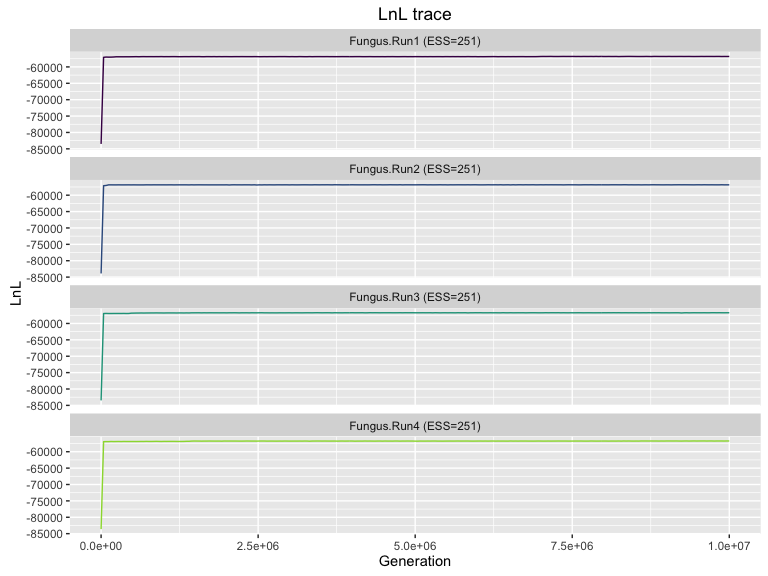``````##
## \$density.plot``````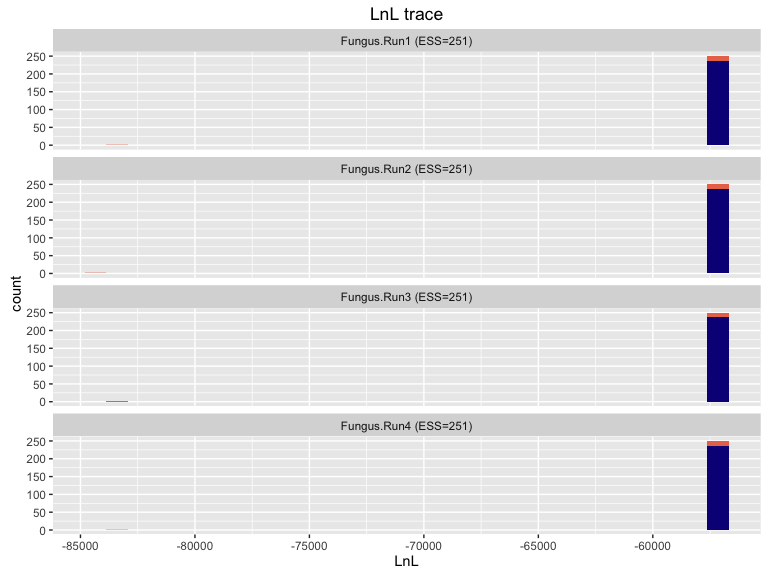Notice that we get two plots here. The trace plot shows us the likelihood of the trees being explored in each generation of the chain. The density plots show the same information in histogram form. The density plots look a little weird because we’ve still got burnin included - they’re trying to plot histograms with a couple of extreme outliers. Let’s fix that by eliminating 50 trees from the start of the chain and trying again.

``makeplot.param(fungus, burnin = 50, "LnL")``
``##  "Creating trace for LnL"``
``## \$trace.plot``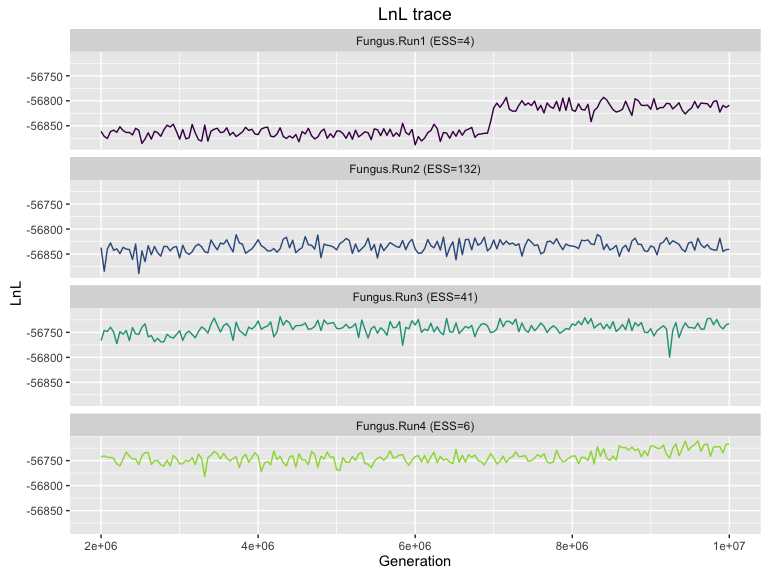``````##
## \$density.plot``````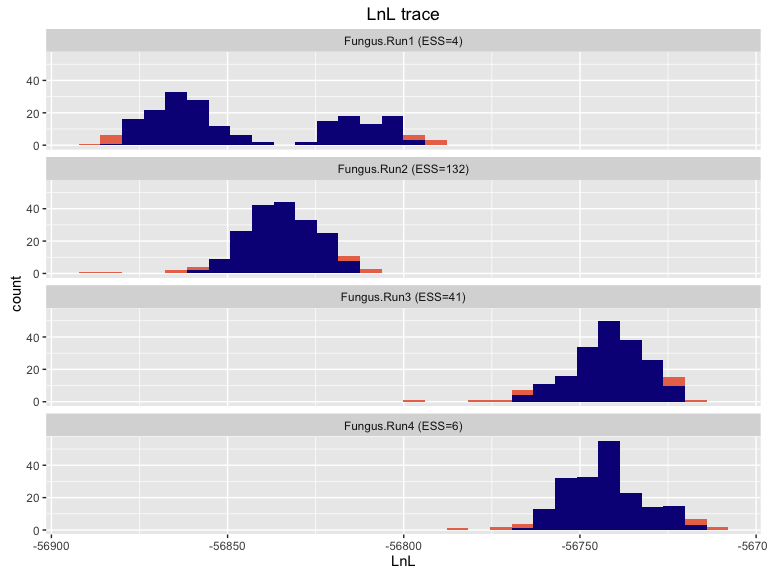Those histograms look a lot more reasonable, but the top likelihood trace plot has a pretty massive jump about seven million generations in. That indicates that that chain probably jumped from one local optimum to another. Notice also that the density plots have a lot of information in them. First off, the coloring represents data values that fall inside and outside the central 95% confidence interval. Notice that the top plot (Run 1) is bimodal. That’s another hint that your chain has jumped between optima somewhere along the line. Even more interesting is the fact that runs 1 and 2 seem to be exploring trees with likelihoods that are quite a bit different from chains 3 and 4 - about 100 LnL difference, in fact. Given that those likelihoods are presented as logs, this is quite a substantial difference. Even though we’ve run four separate chains for ten million generations, we are nowhere near getting a reliable answer from this data.

There’s another indicator here that you should make sure to look at: the ESS figures in the top bar of each plot. These represent the effective samples size for the plotted parameter in a given chain. You can see here that the ESS values for runs 1 and 4 are extremely low; normally you aim for an ESS of over 200 as a rule of thumb.

Let’s compare the salamander plots for makeplot.param. We’ll just eliminate 50 trees as burnin here as well, based on previous experience mucking around with this data.

``makeplot.param(salamanders, burnin = 50, "LnL")``
``##  "Creating trace for LnL"``
``## \$trace.plot``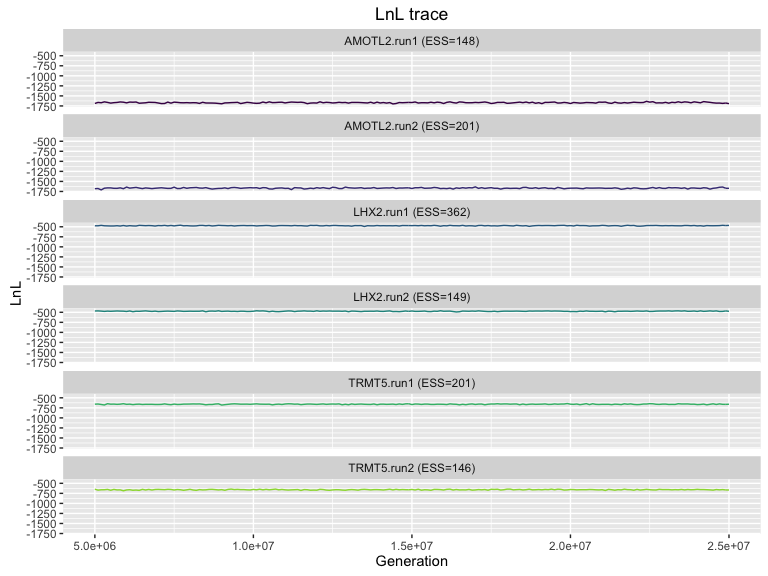``````##
## \$density.plot``````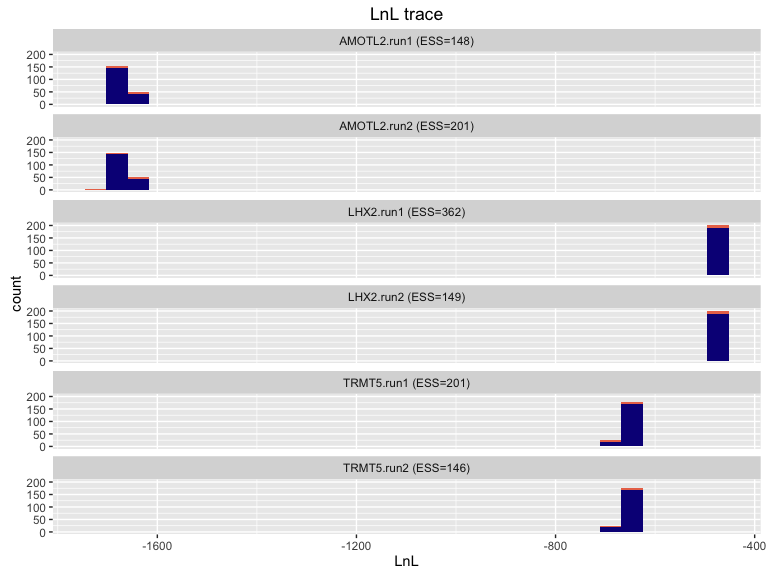Okay let’s pause here to remind ourselves of a key difference between the salamander and fungus data: the fungus data is four separate runs built using the same sequence data. The salamander data, on the other hand, represents three separate bits of sequence data, with two chains for each. As such we should expect all of the fungus chains to look pretty much the same, but the salamander chains should consist of three pairs of very similar chains, assuming all of those chains have reached stationarity. That is in fact what we see here, but the chains are sufficiently different that we’re compressing a lot of variation down just so we can visualize everything at once. Let’s concentrate on just those first two chains, which should be very similar because they are based on the same sequence data. We can do this just by picking the first and second elements out of the salamanders object, which is itself just a regular R list.

``````salamanders.amotl <- list(salamanders[], salamanders[])
makeplot.param(salamanders.amotl, burnin = 50, "LnL")``````
``##  "Creating trace for LnL"``
``## \$trace.plot``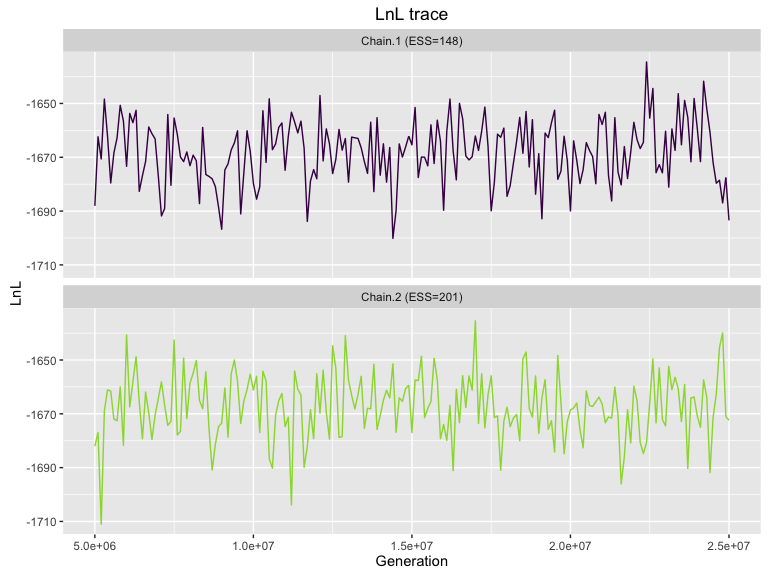``````##
## \$density.plot``````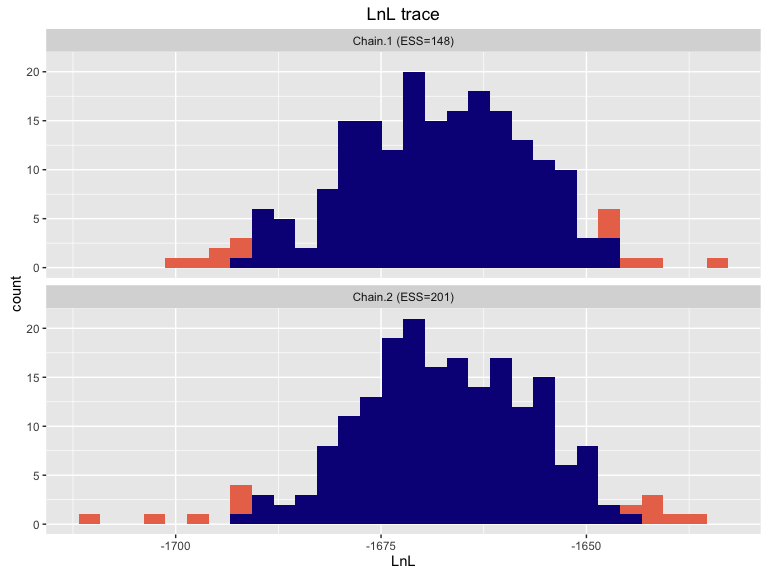Very nice. We can now see the variation in the trace plot, and the histograms look quite comparable. At this zoomed-in scale we’re not necessarily looking for the trace plot to be perfectly flat; we mostly just want to see that there is no long term directional trend.

We can build similar plots with any of the other model parameters in the log table. Here we’ll explore the parameter pi.A.

``makeplot.param(fungus, burnin = 50, "pi.A.")``
``##  "Creating trace for pi.A."``
``## \$trace.plot``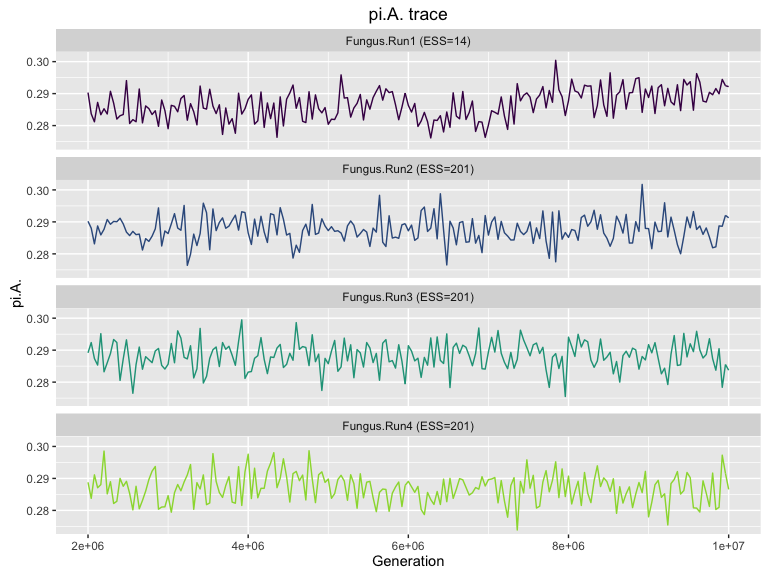``````##
## \$density.plot``````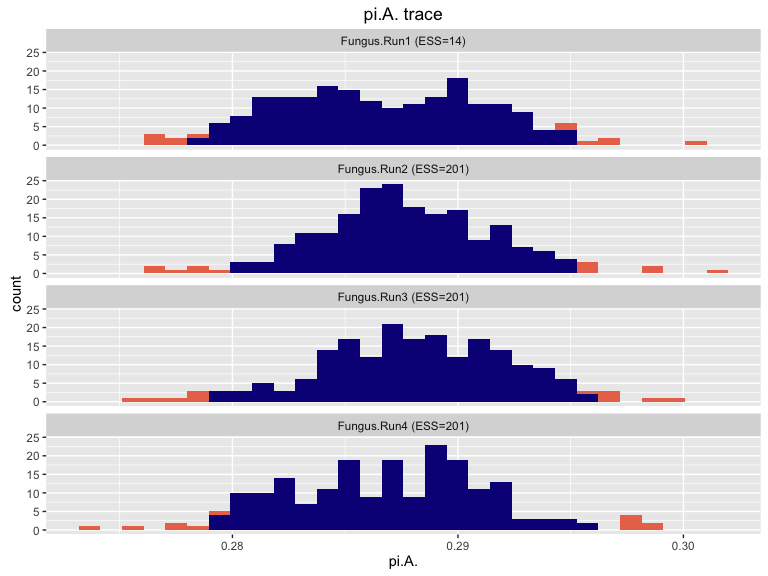It’s worth looking at the difference between the parameter plot here and the likelihood plot above for the same data. Note that the pi.A plots don’t show anywhere near as much of a long term trend as the likelihood plots, and that the ESS for pi.A is higher than for likelihood. Also note how similar the histograms for pi.A are between chains, compared to the level of similarity seen between the likelihood plots. This argues strongly for not relying too heavily on model parameters for estimating chain convergence; the model of molecular evolution is quite likely to reach stationarity long before our topological estimates. However, even those likelihood plots can be far too generous when it comes to diagnosing convergence, as we’ll see below.

## Topology trace plots and histograms

We can also make plots representing tree topology that are very similar to our parameter plots. These plots depict the distance of the chain from a focal tree as a function of generation in the chain. Although these plots are necessarily discarding some variation by dint of compressing an N dimensional tree space to a single axis, they can highlight severe problems with some chains that might not be visible from likelihood plots.

``makeplot.topology(salamanders.amotl, burnin = 50)``
``````##  "Creating trace for tree topologies"
##  "Calculating approximate ESS with sampling intervals from 1 to 100"``````
``## \$trace.plot``